ControlNet

## 最初的Transformer

### 解决并行问题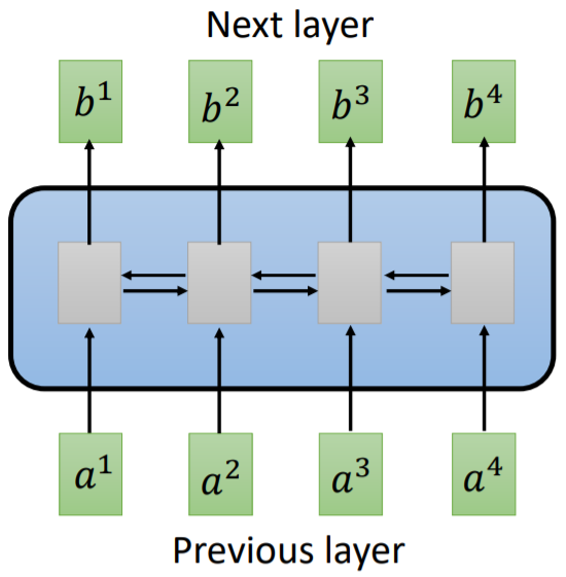Fig. 1. RNN layer. Adapted from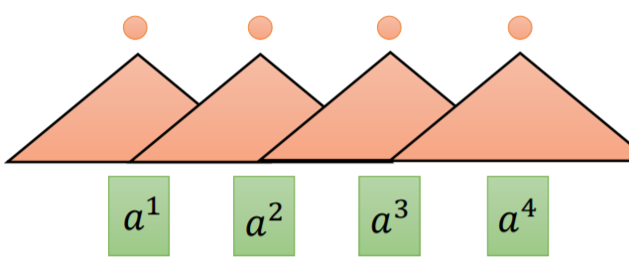Fig. 2. Convolutional layer. Adapted from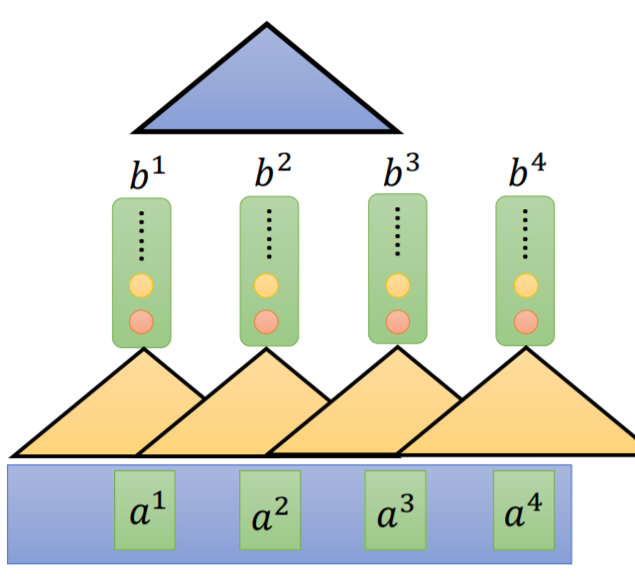Fig. 3. Stacked convolutional layer. Adapted from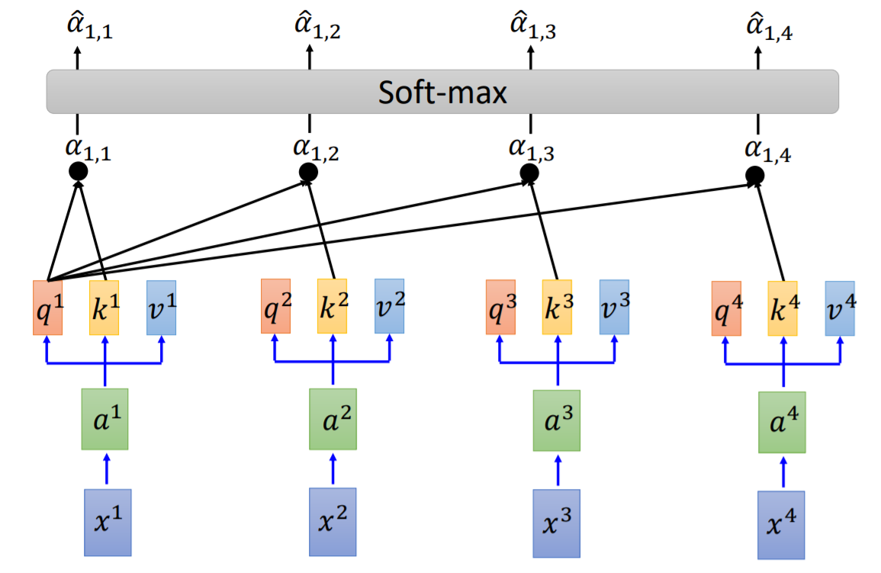Fig. 4. Calculation of $K^i$ and $Q^i$ in self-attention layer. Adapted from 

$$\begin{split} q^i &= W^qa^i \\ k^i &= W^ka^i \\ v^i &= W^va^i \end{split}$$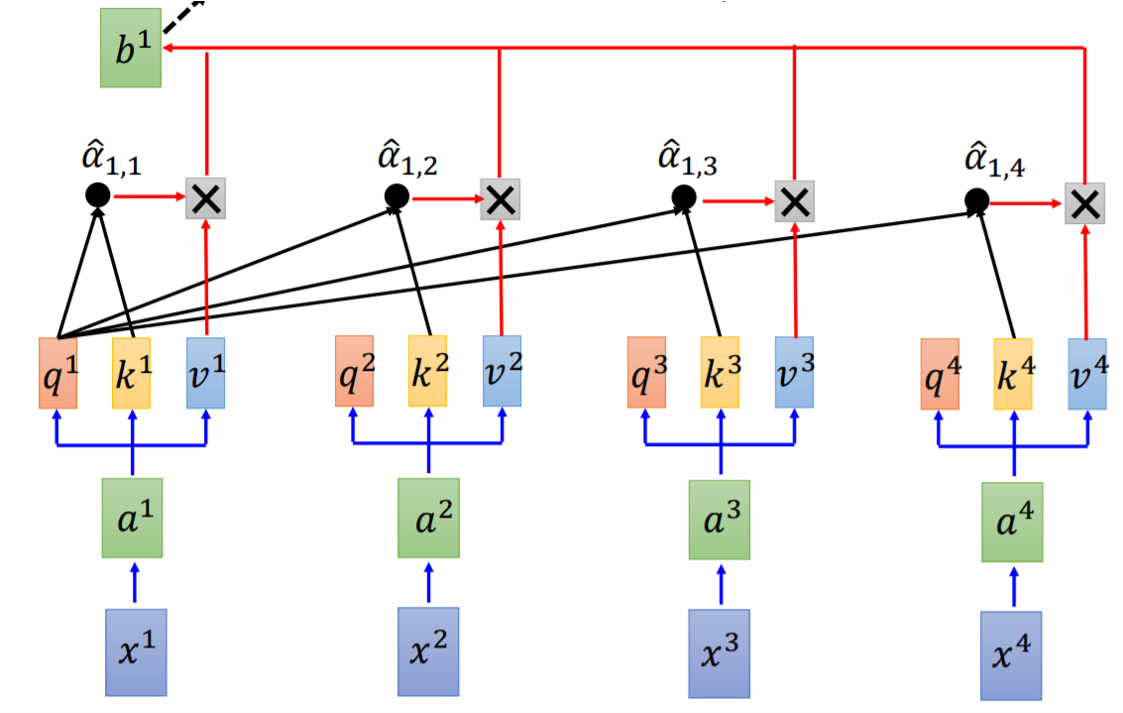Fig. 5. Calculation of $b^i$ in self-attention layer. Adapted from 

$$b^1 = \sum_i\hat{\alpha}_{1,i}v^i$$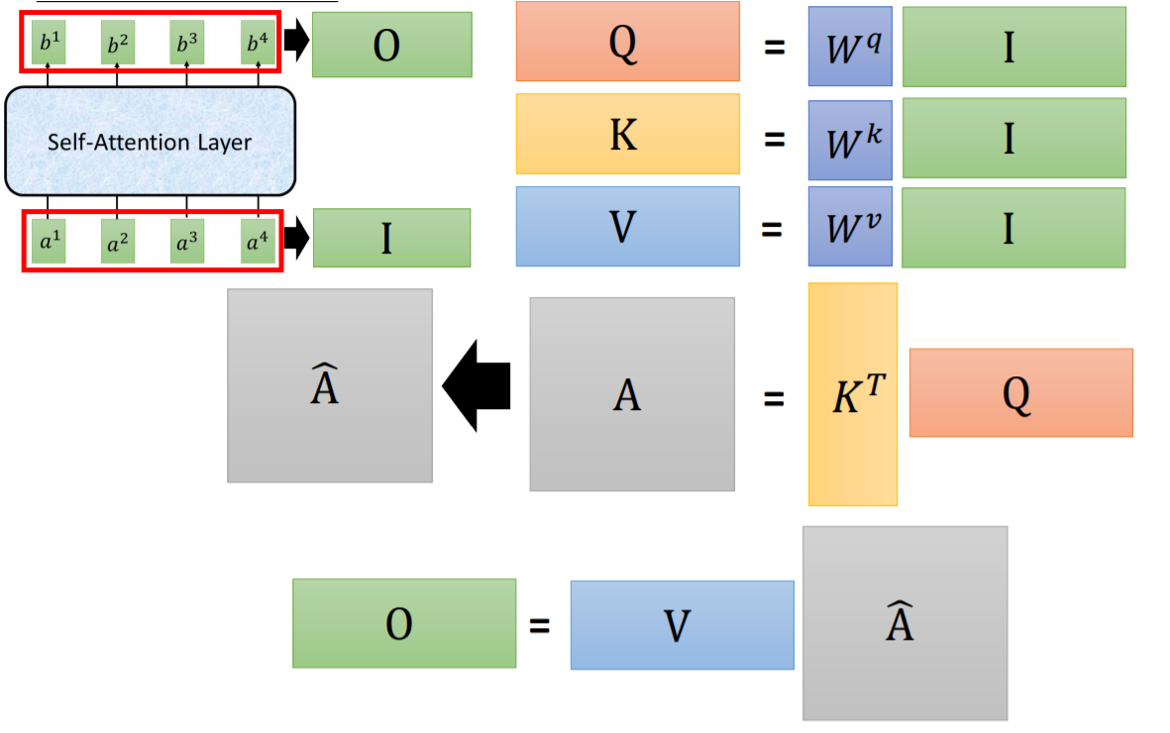Fig. 6. Matrix multiplication form in self-attention layer. Adapted from 

### Transformer结构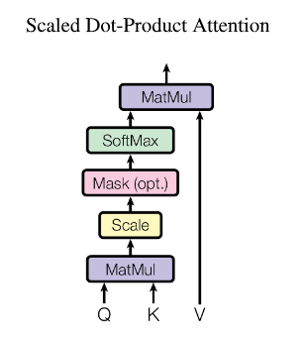Fig. 7. Scaled dot product attention. Adapted from 

$$Attention(Q,K,V)=softmax(\frac{QK^T}{\sqrt{d_K}})V$$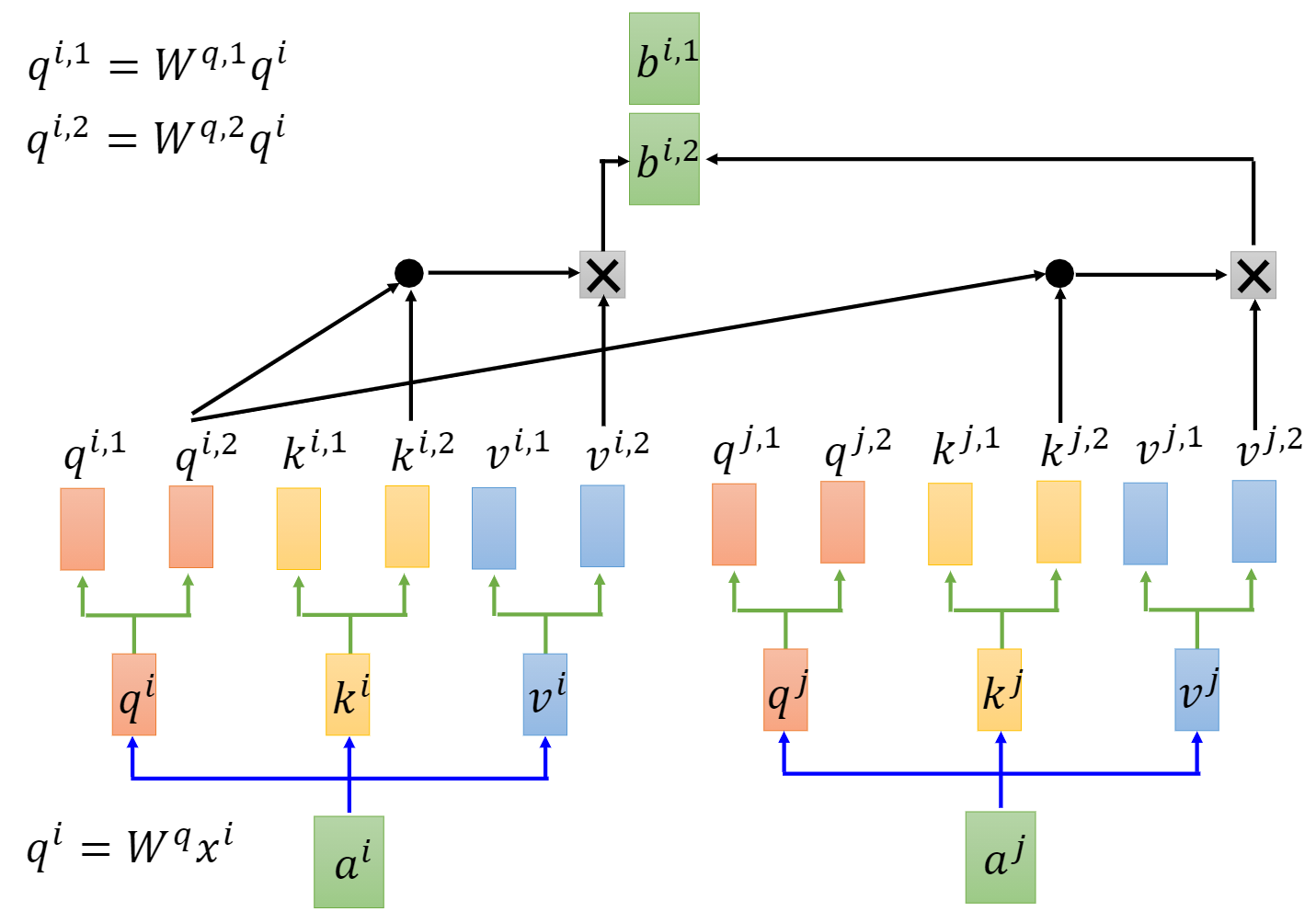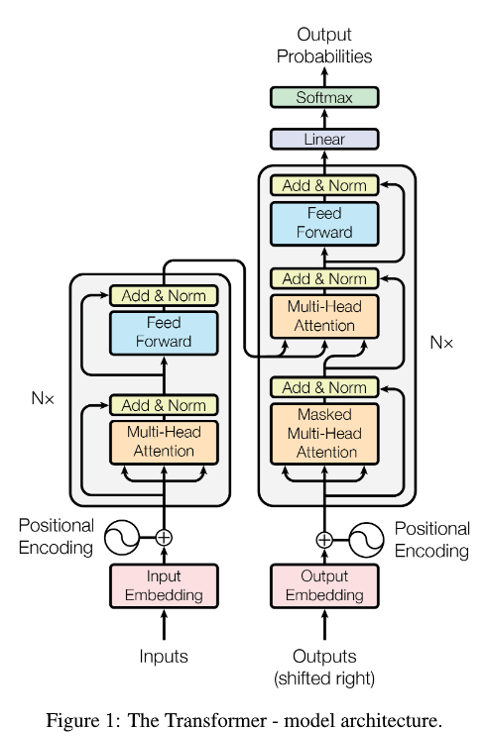Fig. 9. Transformer structure. Adapted from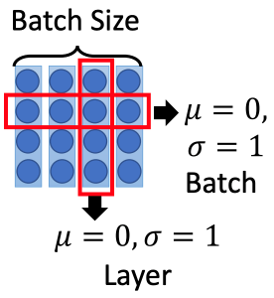Fig. 10. Layer normalization. Adapted from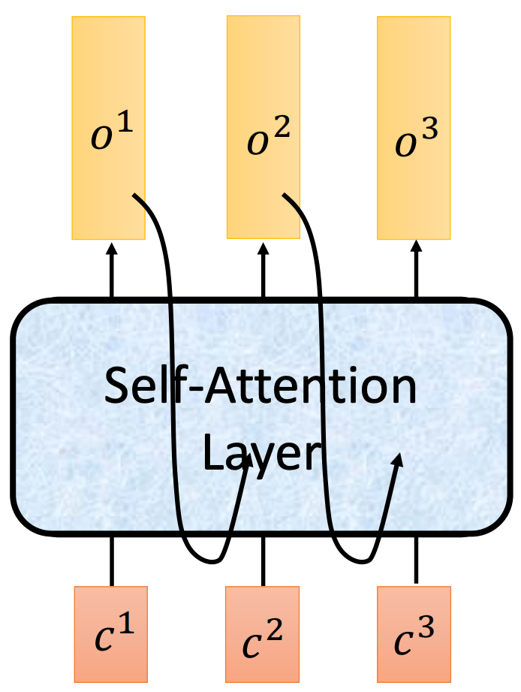Fig. 11. Transformer inference. Adapted from 

## 尝试把Transformer用于CV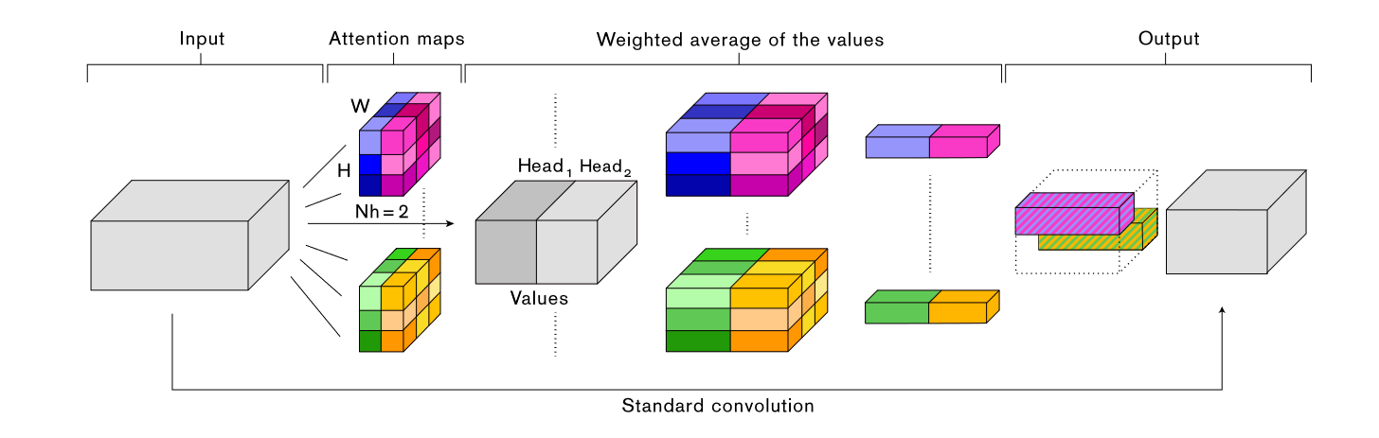Fig. 12. Attention augmented convolutional network. Adapted from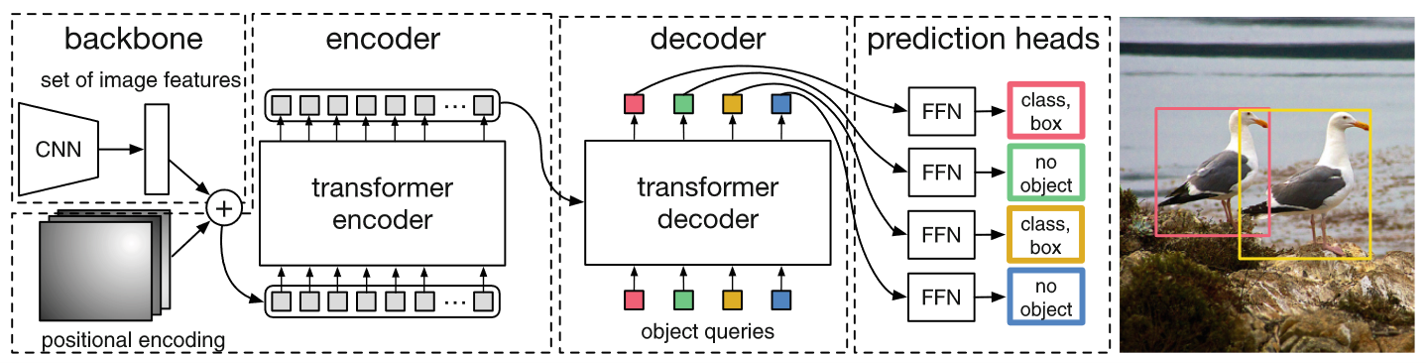Fig. 13. Object detection transformer. Adapted from 

## Vision Transformer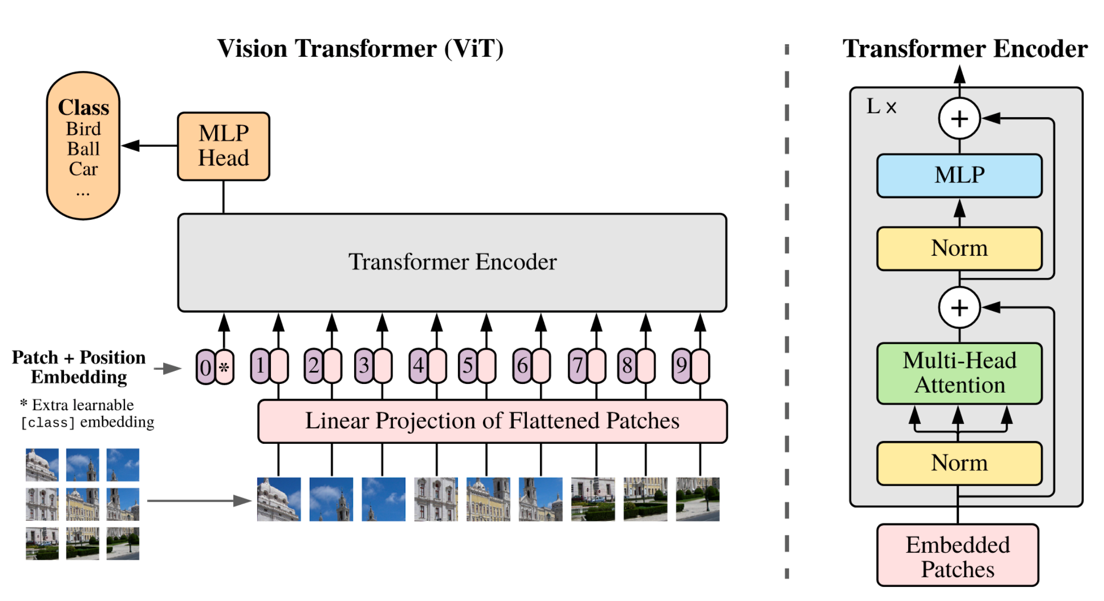Fig. 14. Vision transformer. Adapted from 

### 具体步骤

$$x\in H\times W\times C$$

$$x_p\in N \times (P^{2}C)$$

$$N=\frac{HW}{P^2}$$

$$z_p\in N\times D$$

$$Z=[z_{class},z_p^1,z_p^2,z_p^3,\cdots,z_p^N]+E_{pos}$$

### Class Token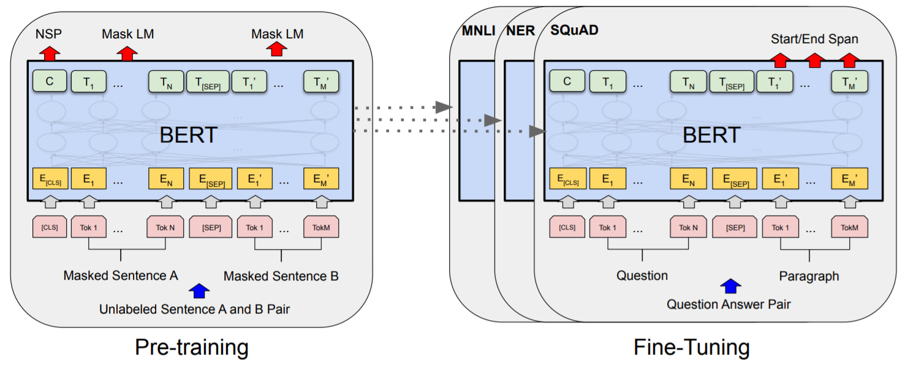Fig. 15. BERT. Adapted from 

### Positional Encoding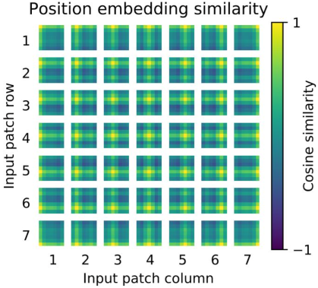Fig. 16. Positional encoding in ViT. Adapted from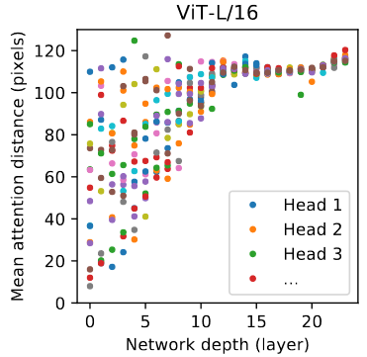Fig. 17. Attention distance. Adapted from 

## Multiscale Vision Transformer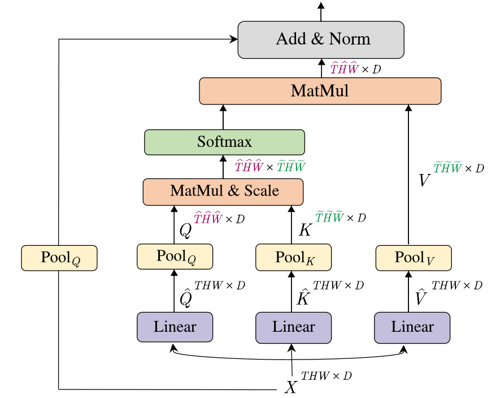Fig. 18. Multiscale vision transformer. Adapted from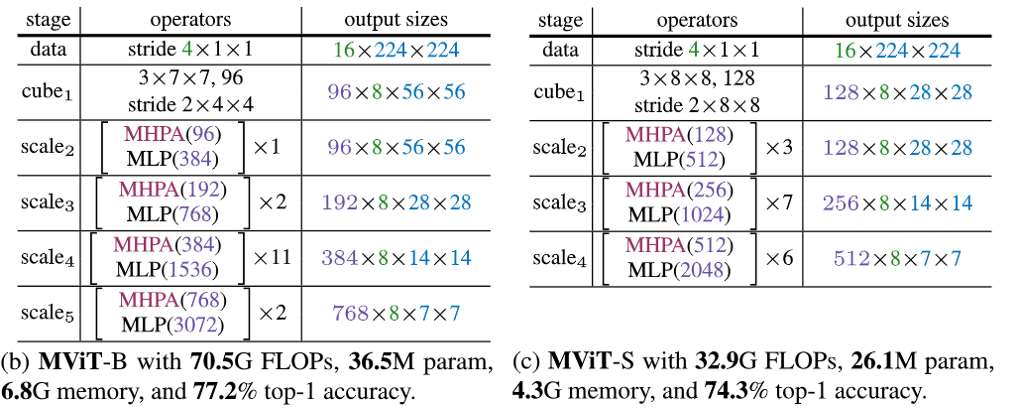Fig. 19. MViT structures. Adapted from 

## 推荐一个GitHub Repository

https://github.com/dk-liang/Awesome-Visual-Transformer

## 参考文献

•  A. Vaswani et al., “Attention is all you need,” in Proceedings of the 31st International Conference on Neural Information Processing Systems, Red Hook, NY, USA, Dec. 2017, pp. 6000–6010.
•  S. Hochreiter and J. Schmidhuber, “Long Short-Term Memory,” Neural Computation, vol. 9, no. 8, pp. 1735–1780, Nov. 1997, doi: 10.1162/neco.1997.9.8.1735.
•  K. Cho et al., “Learning Phrase Representations using RNN Encoder-Decoder for Statistical Machine Translation,” arXiv:1406.1078 [cs, stat], 2014.
•  "Transformer & BERT", Speech.ee.ntu.edu.tw, 2021.
•  Y. Lecun, L. Bottou, Y. Bengio, and P. Haffner, ‘Gradient-based learning applied to document recognition’, Proceedings of the IEEE, vol. 86, no. 11, pp. 2278–2324, Nov. 1998, doi: 10.1109/5.726791.
•  K. He, X. Zhang, S. Ren, and J. Sun, “Deep Residual Learning for Image Recognition,” in Proceedings of the IEEE Conference on Computer Vision and Pattern Recognition, 2016, pp. 770–778.
•  J. L. Ba, J. R. Kiros, and G. E. Hinton, “Layer Normalization,” arXiv:1607.06450 [cs, stat], 2016.
•  S. Ioffe and C. Szegedy, “Batch Normalization: Accelerating Deep Network Training by Reducing Internal Covariate Shift,” in International Conference on Machine Learning, Jun. 2015, pp. 448–456.
•  I. Bello, B. Zoph, A. Vaswani, J. Shlens, and Q. V. Le, “Attention Augmented Convolutional Networks,” in Proceedings of the IEEE/CVF International Conference on Computer Vision, 2019, pp. 3286–3295.
•  N. Carion, F. Massa, G. Synnaeve, N. Usunier, A. Kirillov, and S. Zagoruyko, “End-to-End Object Detection with Transformers,” in Computer Vision – ECCV 2020, Cham, 2020, pp. 213–229, doi: 10.1007/978-3-030-58452-8_13.
•  A. Dosovitskiy et al., “An Image is Worth 16x16 Words: Transformers for Image Recognition at Scale,” arXiv:2010.11929 [cs], Oct. 2020.
•  J. Devlin, M.-W. Chang, K. Lee, and K. Toutanova, “BERT: Pre-training of Deep Bidirectional Transformers for Language Understanding,” in Proceedings of the 2019 Conference of the North American Chapter of the Association for Computational Linguistics: Human Language Technologies, Volume 1 (Long and Short Papers), Minneapolis, Minnesota, Jun. 2019, pp. 4171–4186, doi: 10.18653/v1/N19-1423.
•  H. Fan et al., “Multiscale Vision Transformers,” arXiv:2104.11227 [cs], Apr. 2021.
•  "dk-liang/Awesome-Visual-Transformer", GitHub, 2021. [Online]. Available: https://github.com/dk-liang/Awesome-Visual-Transformer.